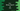# Binary Search in C++ STL (Standard template library)## Binary Search in C++ STL (Standard template library):

Binary search is a searching library used to search a value in a sorted sequence. It is done in divide and search approach.

It starts with the whole sequence and compares the searching element with the middle element of the sequence. If it is smaller than the middle element, it ignores the mid to end division and picks the start to mid division. If it is bigger than the middle, it moves to the right division. Similarly, it keeps dividing the sequence in half to find the number.

Since we are dividing the sequence and comparing the element with the middle element, it is always required a sorted sequence.

We can write the binary search algorithm from scratch or we can use the already defined method in standard template library or STL of C++. It is defined in algorithm header and called binary_search.

binary_search is defined as below:

``bool binary_search(ForwardIterator first, ForwardIterator last, const T& val, Compare comp)``

Here,

• first is the Forward Iterator for the first element in the sorted sequence.
• last is the Forward Iterator for the last element in the sorted sequence.
• val is the value to search in the sequence.
• comp is a function that accepts two values of same type as other elements in the sequence and returns one boolean that defines whether the first value should go before the second value. This function should not modify any element in the sequence.

This method returns one boolean value. It returns true if the value provided is found in the sequence or false if it is not found.

### C++ program(for sorted array):

Below is the complete C++ program:

``````#include<iostream>
#include<algorithm>

using namespace std;

bool comparator(int first, int second){
return first<second;
}

int main(){
int givenValues[] = {1,3,5,7,9,11,14,17,19,23,25,27,33};
int arrSize = sizeof(givenValues)/sizeof(givenValues);

int valueToFind;
cout<<"Enter a number to find :"<<endl;
cin>>valueToFind;

bool found = std::binary_search(givenValues, givenValues + arrSize, valueToFind, comparator);

if(found){
cout<<"It is found in the array"<<endl;
}else{
}
return 0;
}``````

### Explanation:

• This program is for a already sorted array. Here, givenValues is the given array and it is sorted.
• arrSize variable is used to store the size of the array.
• valueToFind variable is used to hold the number that we want to find in the array. This value we are taking from the user as input.
• We are calling biinary_search with the arguments as we defined before. comparator is the function that compares two integer values and returns one boolean. It returns true if the first value is smaller. Else, it returns false. The return boolean of binary_search is stored in the variable found.
• Based on the value of found, we are printing one message if the value is found in the array or not.

### Sample output:

Below are sample outputs of the above program:

``````Enter a number to find :
33
It is found in the array

Enter a number to find :
322

Enter a number to find :
11
It is found in the array``````

## Program for unsorted array:

If the array is not sorted, we need to sort the array first. We can do it as like below :

``````int givenValues[] = {11,2,4,55,1,0};

int arrSize = sizeof(givenValues)/sizeof(givenValues);
sort(givenValues, givenValues + arrSize);``````

You can add the sort function call to sort the values before calling binary_search.